EXERCISE 4.2.

1) Classify the following curves as (i) Open or (ii) Closed

a)  Open                                                                  b)   Closed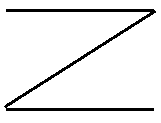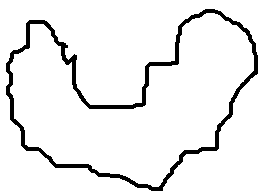(c)   Open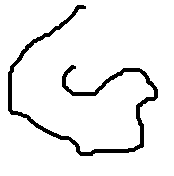d)                                                                     e)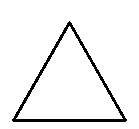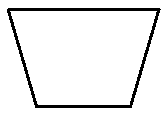Closed                                                        Closed

2) Draw rough diagrams to illustrate the following:
a) Open curve                 b) Closed curve
Ans:
a)b)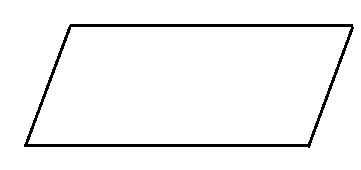3) Draw any polygon and shade its interior.

Ans.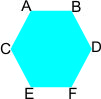∴ ABCDEF  is the required polygon

4) Consider the given figure and answer the questions:
a) Is it a curve

Ans: Yesb) Is is closed?
Ans: Yes

5) Illustrate, if possible, each one of the following with a rough diagram:
a) A closed curve that is not a polygon
Ans: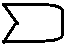b) An open curve made up entirely of line segments.
Ans: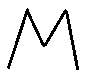c) A polygon with two sides
Ans: Not possible